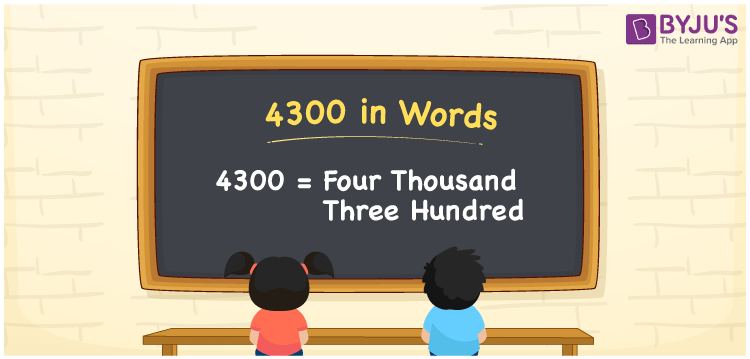# 4300 in Words

4300 in words is written as Four thousand three hundred. In both the International System of Numerals and the Indian System of Numerals, 4300 is written as Four thousand three hundred. The number 4300 is a Cardinal Number as it represents some quantity, “how many of something” or “how much of something”. For example, “that electronic gadget costs 4300 rupees”.

 4300 in words Four thousand three hundred Four thousand three hundred in Number 4300

## 4300 in English Words

4300 in English words is read as “Four thousand three hundred.”## How to Write 4300 in Words?

To write 4300 in words, we shall use the place value chart. In the place value chart, put 4 in the thousands, 3 in the hundreds place and 0 in the rest. Let us make a place value chart to write the number 4300 in words.

 Thousands Hundreds Tens Ones 4 3 0 0

Thus, we can write the expanded form as

4 × Thousand + 3 × Hundred + 0 × Ten + 0 × One

= 4 × 1000 + 3 × 100 + 0 × 10 + 0 × 1

= 4000 + 300 + 0 + 0

= 4300

= Four thousand three hundred.

4300 is the natural number between 4299 and 4301.

4300 in words – Four thousand three hundred

• Is 4300 an odd number? – No
• Is 4300 an even number? – Yes
• Is 4300 a perfect square number? – No
• Is 4300 a perfect cube number? – No
• Is 4300 a prime number? – No
• Is 4300 a composite number? – Yes

## Frequently Asked Questions on 4300 in Words

Q1

### How to write 4300 in words?

4300 in words is written as Four thousand three hundred.
Q2

### How to write 4300 in words in the International and Indian System of Numerals?

In both, the system of numerals, 4300 in words, is written as Four thousand three hundred.
Q3

### How to write 4300 in a place value chart?

In the place value chart, write 4 in the thousands, 3 in the hundreds and 0 in the tens and ones, respectively.